Open-File Report 2005-1413

### Flightline Processing

#### Flightline Data in the United States

The flightline data in the United States (US) were taken from Duval (2001), Duval and Riggle (1999), and Duval (2000) and were compiled into five separate databases that cover the eastern US, east central US, west central US, western US, and Alaska. These surveys are organized by the 1:250,000 scale quadrangles of the US. In the databases, the data are identified by line numbers and flight numbers. Flight numbers were assigned to the surveys in an arbitrary sequence and the number assigned to a particular survey can be determined by accessing the index maps of the surveys.

The flightline data were previously processed by Duval (2001) and Duval and Riggle (1999) to produce internally consistent data and they describe the procedures that they used. After compilation into the databases, grids of the data were calculated using a grid interval of 2 km and a minimum-curvature algorithm similar to that described by Briggs (1974). A subjective visual examination of the data was made to determine whether any of the surveys required further corrections. For a limited number of surveys, corrections were applied either to the entire survey or to individual flightlines within a survey. In all cases the corrections applied were to multiply the data by a constant and the magnitude of the corrections applied were generally less than 20 percent. Decisions to apply corrections were based upon obvious discontinuities along survey boundaries or along individual flightlines. Click here to view an example of flightlines requiring individual corrections. Final grids for each of the databases were inserted into grids of the United States and Canada. Overlap between adjacent grids ensured the elimination of possible discontinuities between grids.

The flightline data in Canada were taken from data sets compiled and published at scales of 1:250,000 and 1:1,000,000. These data were processed by the Radiation Geophysics Section of the Geological Survey of Canada and grids of the data were calculated using a grid interval of 2 km and a minimum-curvature algorithm. Click here to view a list of the references for the various data sets.

### Calculation of Terrestrial Absorbed Dose

The absorbed dose from gamma-rays emitted as a result of the radioactive decay of potassium, uranium, and thorium, and their daughter products in rocks and soils was calculated using the equation

### D = 13.2 K + 5.48 eU + 2.72 eTh

where K is the concentration of potassium in units of percent K, eU is the concentration of uranium in ppm, eTh is the concentration of thorium in ppm, and D is the absorbed dose in nanoGrays per hour (nGy/hr). This equation is based upon the equation published by Grasty and others (1984). The grid of gamma-ray absorbed dose was calculated using the grids of potassium, uranium, and thorium concentrations. Click here to view a map of the absorbed dose.

### Calculation of Cosmic-ray Absorbed Dose as a Function of Elevation

The cosmic-ray absorbed dose was calculated as a function of digital elevation data using equations published by Boltneva, Nazarov, and Fridman (1974). The absorbed dose calculated here does not include ionization caused by neutrons. The general equation can be written as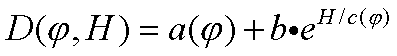where D is the absorbed dose in units of mrad/yr, f is the latitude (degrees), H is the elevation (km), b is a constant, and a and c are functions of the latitude.  The grid of elevations was used to calculate the cosmic-ray absorbed dose and the values were converted to units of nanoGrays per hour (nGy/hr). Click here to view a map of the cosmic-ray absorbed dose.

Equations used for b, a, and c are given below.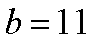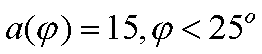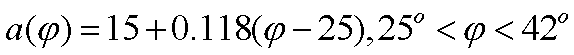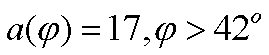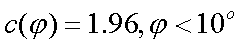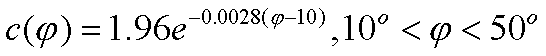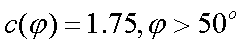Home | Data Files | Data Processing | Flightline Databases | Maps | References | Glossary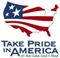U.S. Department of the Interior | U.S. Geological Survey
URL: http://pubsdata.usgs.gov/pubs/of/2005/1413/dataproc.htm
Page Contact Information: USGS Publications Team